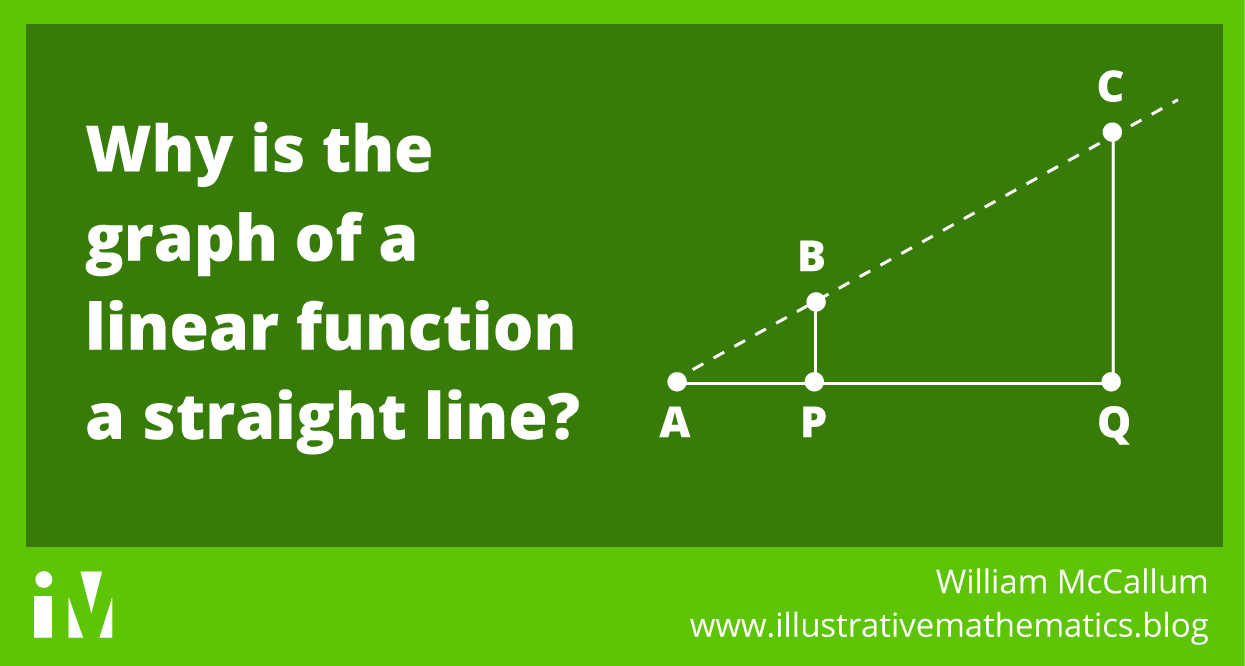## Why is the graph of a linear function a straight line?

By William McCallum In my last post I wrote about the following standard, and mentioned that I could write a whole blog post about the first comma. 8.F.A.3. Interpret the equation $y = mx + b$ as defining a linear function, whose graph is a straight line; give...